# Pagoda2 Walkthrough

## Overview

This walkthrough will guide you through the analysis of single-cell RNA-seq with pagoda2.

Pagoda2 performs basic tasks such as cell size normalization/corrections and residual gene variance normalization, and can then be used to perform tasks such as identifying subpopulations and running differential expression within individual samples. The companion web application allows users to interactively explore the transcriptional signatures of subpopulations within the dataset. Users are able to investigate the molecular identity of selected entities, and inspect the associated gene expression patterns through annotated gene sets and pathways, including Gene Ontology (GO) categories. Users may also perform differential expression of selected cells via the frontend application.

We will begin by showing the quickest way to process data with pagoda2, using the function `basicP2proc()`. We will then systematically re-run this analysis step-by-step, beginning with loading the dataset and performing QC. This will more thoroughly detail and motivate the steps involved in quality control/processing. Finally we will generate an interactive web application in order to explore the dataset.

## I. Fast Processing and Exploration with Pagoda2

This is the rapid walkthrough of pagoda2, showing how the package allows users to quickly process their datasets and load them into an interactive frontend application.

``````library(Matrix)
library(igraph)
library(pagoda2)
library(dplyr)
library(ggplot2)``````

We have pre-generated a dataset of 3000 bone marrow cells that you can load as a matrix directly using the package `p2data`, which is available through a `drat` repository on GitHub. Note that the size of the ‘p2data’ package is approximately 6 MB. This package may be installed as follows:

``install.packages('p2data', repos='https://kharchenkolab.github.io/drat/', type='source')``

(Please see the drat documentation for more comprehensive explanations and vignettes regarding `drat` repositories.)

The following command load the dataset of 3000 bone marrow cells as a sparse matrix:

``countMatrix <- p2data::sample_BM1``

Note that many users will wish to read in their own data from the outputs of the 10x preprocessing pipeline CellRanger, i.e. the gzipped tsv files of matrices, features, and barcodes. For this, we have provided the function `read10xMatrix()`.

Next we feed this input into the function `basicP2proc()`, which performs all basic pagoda2 processing. That is, the function will adjust the variance, calculate PCA reduction, make a KNN graph, identify clusters by multilevel optimization (the Louvain algorithm), and generate largeVis and tSNE embeddings.

``````## load the dataset
countMatrix <- p2data::sample_BM1
## all basic pagoda2 processing with basicP2proc()
p2.processed <- basicP2proc(countMatrix, n.cores=1, min.cells.per.gene=10,
n.odgenes=2e3, get.largevis=FALSE, make.geneknn=FALSE)``````
``````## creating space of type angular done
## building index ... done
## querying ... done``````

We can now quickly view the results via the interactive web application. First we run `extendedP2proc()` to calculate pathway overdispersion for a specific organism using GO. We currently support three organisms: ‘hs’ (Homo Sapiens), ‘mm’ (Mus Musculus, mouse) or ‘dr’ (Danio Rerio, zebrafish). (This can take some time to run, so we’ll omit it for the vignettes.) Then we create a pagoda2 “web object” to be used for the application. This can be accessed via your web browser with the function `show.app()`.

``````## calculate pathway overdispersion for human
## ext.res <- extendedP2proc(p2.processed, organism = 'hs')

## create app object
## p2app <- webP2proc(ext.res\$p2, title = 'Quick pagoda2 app', go.env = ext.res\$go.env)

## open app in the web browser via R session
## show.app(app=p2app, name='pagoda2 app')``````

And that’s it! You will now be able to interact with the processed dataset via the web browser. The more in-depth demo regarding the web application can be found here.

## II. In-Depth Processing and Analysis

We now will re-run and explain each step within `basicP2proc()`, starting from the beginning:

``````library(Matrix)
library(igraph)
library(pagoda2)
library(dplyr)
library(ggplot2)``````

For the purposes of this walkthrough, we have pre-generated a dataset of 3000 bone marrow cells that you can load as a matrix directly. The following command load the data as a sparse matrix and checks its size:

``````cm <- p2data::sample_BM1
dim(cm)``````
``##  33694  3000``

We see that the matrix has 33k rows and 3k columns. Next let’s have a look at our matrix to see what is in it. We see that genes are named using common gene names and columns by cell barcode.

``cm[1:3, 1:3]``
``````## 3 x 3 sparse Matrix of class "dgCMatrix"
##              MantonBM1_HiSeq_1-TCTATTGGTCTCTCGT-1
## RP11-34P13.3                                    .
## FAM138A                                         .
## OR4F5                                           .
##              MantonBM1_HiSeq_1-GAATAAGTCACGCATA-1
## RP11-34P13.3                                    .
## FAM138A                                         .
## OR4F5                                           .
##              MantonBM1_HiSeq_1-ACACCGGTCTAACTTC-1
## RP11-34P13.3                                    .
## FAM138A                                         .
## OR4F5                                           .``````

We can get more information about how the matrix is stored by running `str()`. To find out more information about the sparse matrix format, check the documentation of the ‘Matrix’ package.

``str(cm)``
``````## Formal class 'dgCMatrix' [package "Matrix"] with 6 slots
##   ..@ i       : int [1:2613488] 33 45 72 153 353 406 436 440 457 484 ...
##   ..@ p       : int [1:3001] 0 864 1701 2607 3256 3856 4537 5271 6030 7002 ...
##   ..@ Dim     : int [1:2] 33694 3000
##   ..@ Dimnames:List of 2
##   .. ..\$ : chr [1:33694(1d)] "RP11-34P13.3" "FAM138A" "OR4F5" "RP11-34P13.7" ...
##   .. ..\$ : chr [1:3000(1d)] "MantonBM1_HiSeq_1-TCTATTGGTCTCTCGT-1" "MantonBM1_HiSeq_1-GAATAAGTCACGCATA-1" "MantonBM1_HiSeq_1-ACACCGGTCTAACTTC-1" "MantonBM1_HiSeq_1-TCATTTGGTACGCTGC-1" ...
##   ..@ x       : num [1:2613488] 1 1 1 9 1 3 1 2 2 20 ...
##   ..@ factors : list()``````

In order to catch outliers, we can begin with a fairly basic procedure of looking at the dependency between the number of molecules measured per cell and the number of genes per cell. Let’s plot the distribution of molecules per cell and molecules per gene for this dataset in log10 scale:

``````old_par <- par(mfrow=c(1,2), mar = c(3.5,3.5,2.0,0.5), mgp = c(2,0.65,0), cex = 1.0)
on.exit(par(old_par))
hist(log10(colSums(cm)+1), main='molecules per cell', col='cornsilk', xlab='molecules per cell (log10)')``````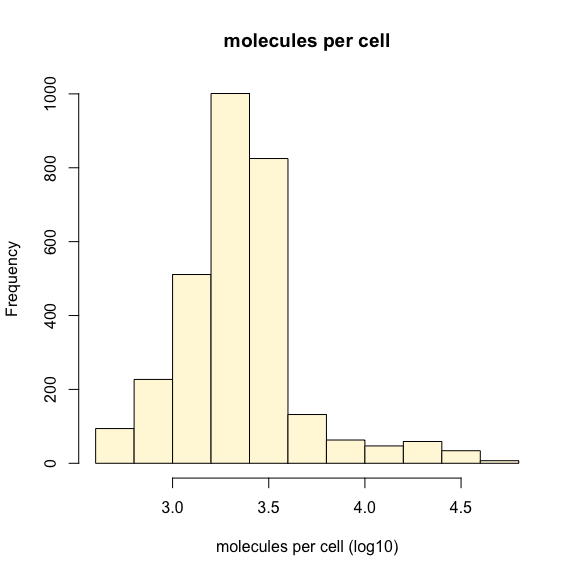``hist(log10(rowSums(cm)+1), main='molecules per gene', col='cornsilk', xlab='molecules per gene (log10)')``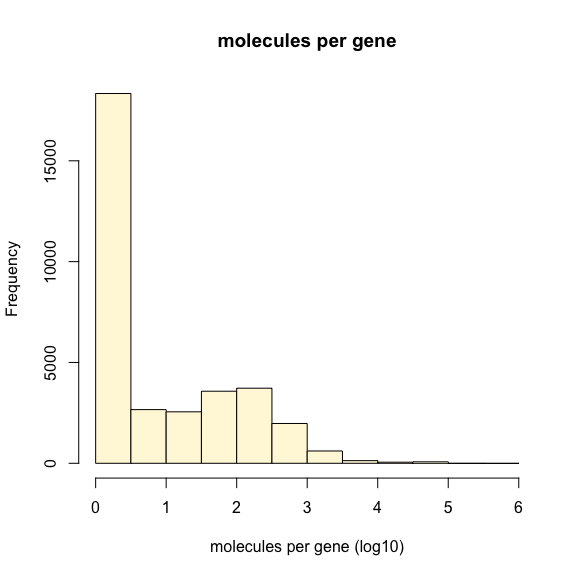This dataset has already been filtered for low quality cells, so we don’t see any cells with fewer that 10^3 UMIs. We can still use the default QC function `gene.vs.molecule.cell.filter()` to filter any cells that don’t fit the expected detected gene vs molecule count relationship. In this case we filter out only 2 cells.

``counts <- gene.vs.molecule.cell.filter(cm, min.cell.size=500)``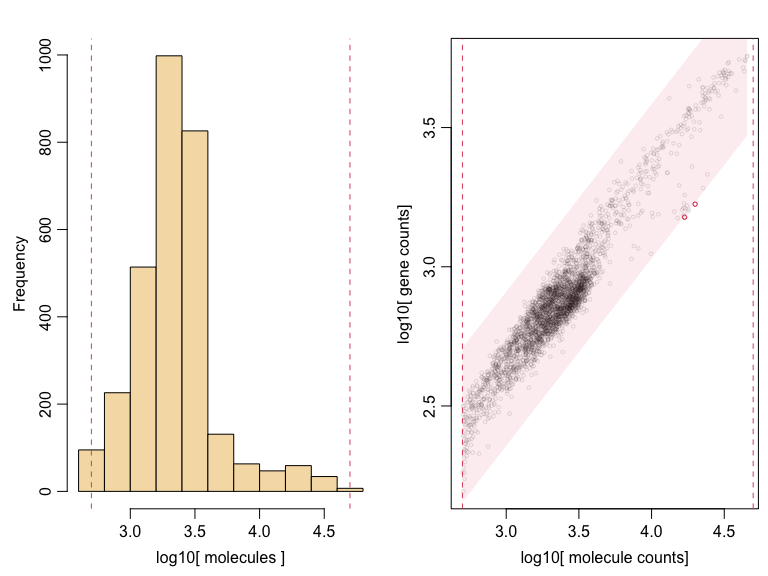Next thing we want to do is to find lowly expressed genes and remove them from the dataset. (Subsequent pagoda2 steps will do this automatically for extremely lowly expressed genes anyway, but for the purpose of this tutorial, we demonstrate this.)

``````hist(log10(rowSums(counts)+1), main='Molecules per gene', xlab='molecules (log10)', col='cornsilk')
abline(v=1, lty=2, col=2)``````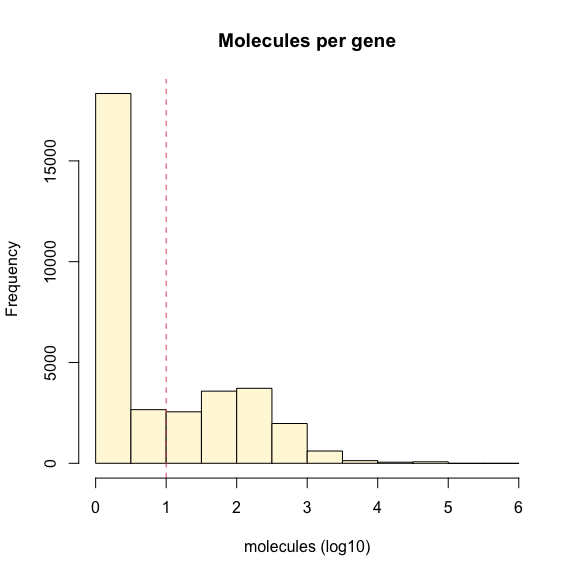Let’s filter out counts less than 10 and check the size of the resulting matrix:

``````counts <- counts[rowSums(counts)>=10, ]
dim(counts)``````
``##  12693  2998``

### Part 2: Analysing Data with Pagoda2

We see that we now have 12k genes and 2998 cells. We are now ready to analyze our data with Pagoda2. Remember: all of the following steps can be done with just two functions automatically (see above) but for the purposes of this tutorial we will go over them step by step to understand what we are doing in more detail. Doing these steps manually also allows us to tune parameters.

First we will generate a pagoda2 object that will contain all our results. Our input matrix contains duplicated gene names (usually originating from different transcripts in the counting process). The easier way to resolve this problem is by making the gene names unique:

``````rownames(counts) <- make.unique(rownames(counts))
r <- Pagoda2\$new(counts, log.scale=TRUE, n.cores=1)``````
``## 2998 cells, 12693 genes; normalizing ...``
``## Using plain model``
``## log scale ...``
``## done.``

Check that you have the matrix in the correct orientation and that number of cells you are getting here is what you expect (like we do here). The input matrix must be in the genes by cells configuration.

Next, we’ll adjust the variance with `adjustVariance()` in order to normalize the extent to which genes with (very) different expression magnitudes will contribute to the downstream analysis.

In order to motivate what we are doing by variance normalization, recall that our goal is to measure the variance of a given gene. (Remember: we are looking at the variation of this gene across the population of cells measured.) The key dependency of this variance is the magnitude. If you thus observe highly-expressed genes, these will always give you high expression variance, regardless of whether these are specific to a cell subpopulation or not.

For variance normalization, we begin by fitting a smooth linear model of variance by magnitude for the dataset. We then quantify the deviation against this dataset-wide trend, and rescale the variance to put the genes on a comparable scale for downstream analysis.

``r\$adjustVariance(plot=TRUE, gam.k=10)``
``## calculating variance fit ...``
``##  using gam``
``## 187 overdispersed genes ... 187``
``## persisting ...``
``##  using gam``
``## done.``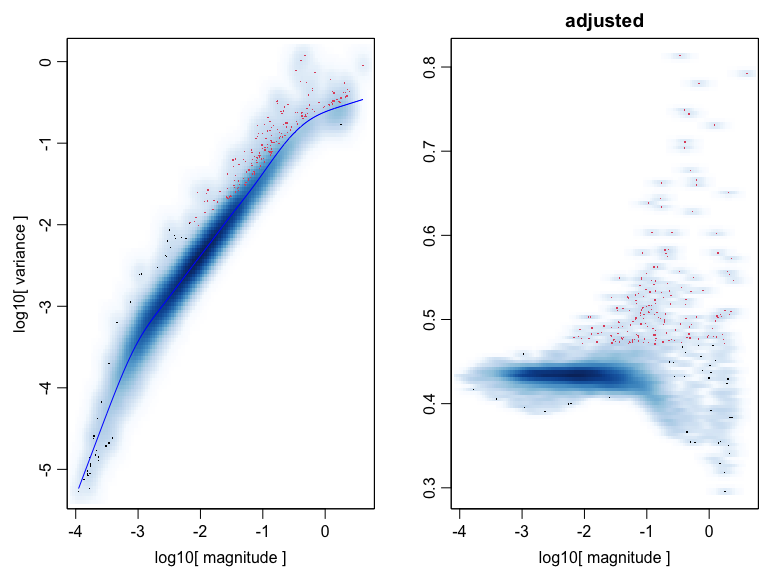Now that the variance of the gene expression is on a comparable scale, there are many alternative ways of proceeding with the downstream analysis. Below we’ll use the simplest default scenario, whereby we first reduce the dataset dimensions by running PCA, and then move into the k-nearest neighbor (KNN) graph space for clustering and visualization calculations.

First, we generate the PCA reduction. Depending on the complexity of the dataset you are analyzing, you may want to adjust the parameter `nPcs`.

``r\$calculatePcaReduction(nPcs=50, n.odgenes=3e3)``
``## running PCA using 3000 OD genes .``
``````## .
## .
## .``````
``##  done``

We will now construct a KNN graph space that will allow us to identify clusters of cells:

``r\$makeKnnGraph(k=40, type='PCA', center=TRUE, distance='cosine')``
``````## creating space of type angular done
## building index ... done
## querying ... done``````

On the basis of this KNN graph, we will call clusters

``r\$getKnnClusters(method=infomap.community, type='PCA')``

Next we generate a 2D embedding of the data with largeVis for visualization:

``````M <- 30
r\$getEmbedding(type='PCA', embeddingType = 'largeVis', M=M, perplexity=30, gamma=1/M)``````
``## Estimating embeddings.``

(Note that largeVis is much faster that the tSNE, which often used in single-cell analysis.)

We now visualize the data:

``r\$plotEmbedding(type='PCA', show.legend=FALSE, mark.groups=TRUE, min.cluster.size=50, shuffle.colors=FALSE, font.size=3, alpha=0.3, title='clusters (largeVis)', plot.theme=theme_bw() + theme(plot.title = element_text(hjust = 0.5)))``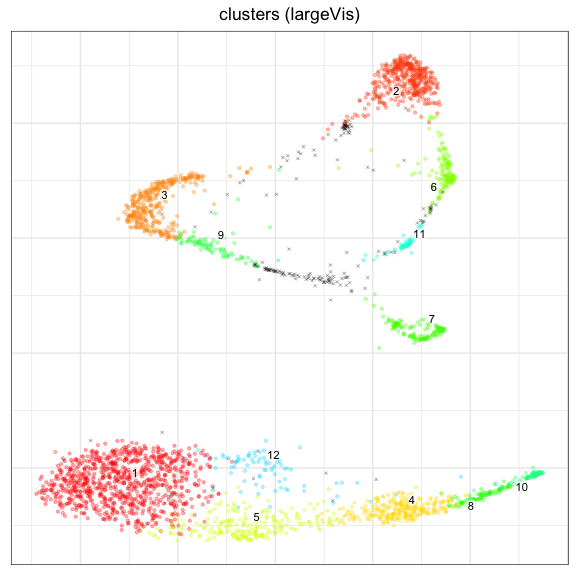We next can constructr and plot a tSNE embedding. (This can take some time to complete.)

``````r\$getEmbedding(type='PCA', embeddingType='tSNE', perplexity=50,verbose=FALSE)
r\$plotEmbedding(type='PCA', embeddingType='tSNE', show.legend=FALSE, mark.groups=TRUE, min.cluster.size=1, shuffle.colors=FALSE, font.size=3, alpha=0.3, title='clusters (tSNE)', plot.theme=theme_bw() + theme(plot.title = element_text(hjust = 0.5)))``````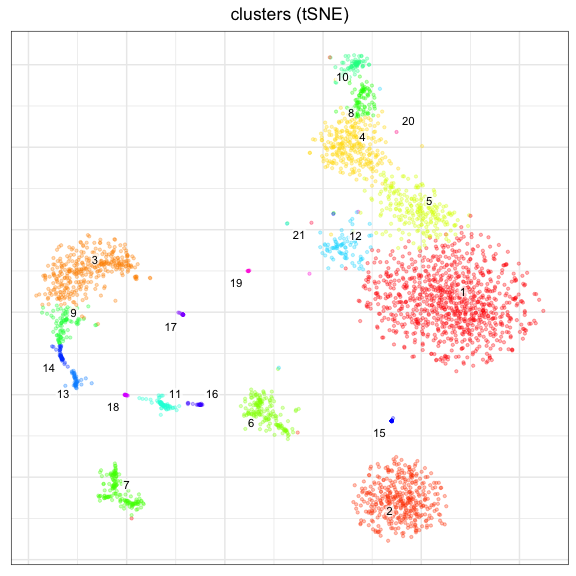Note that we are overlay the expresssion of specific marker genes on this embedding to identify clusters. For instance, subsetting by `"HBB"` will identify heme cells:

``````gene <-"HBB"
r\$plotEmbedding(type='PCA', embeddingType='tSNE', colors=r\$counts[,gene], shuffle.colors=FALSE,
font.size=3, alpha=0.3, title=gene, plot.theme=theme_bw() + theme(plot.title = element_text(hjust = 0.5)))``````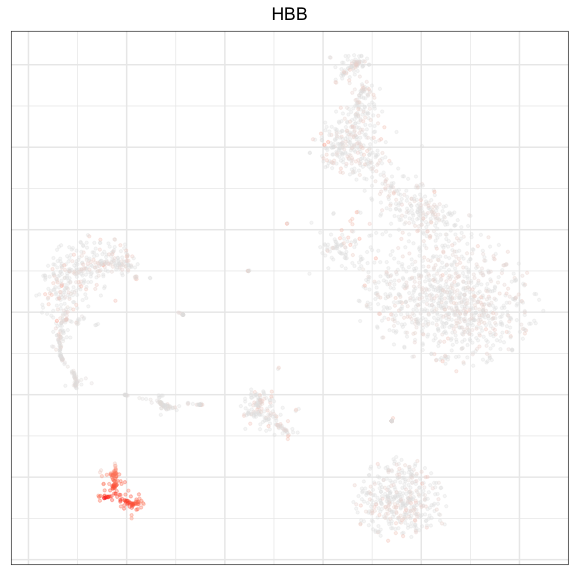Similarly, subsetting by the marker gene `"LYZ"` should show us CD14+ Monocytes:

``````gene <-"LYZ"
r\$plotEmbedding(type='PCA', embeddingType='tSNE', colors=r\$counts[,gene], shuffle.colors=FALSE,
font.size=3, alpha=0.3, title=gene, plot.theme=theme_bw() + theme(plot.title = element_text(hjust = 0.5)))``````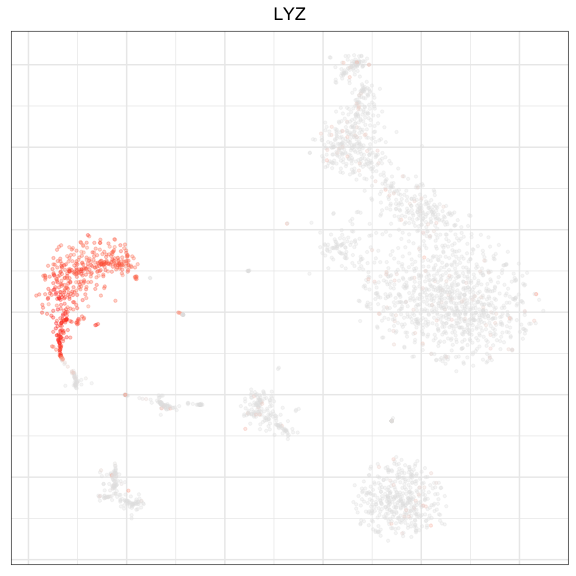Pagoda2 allows us to generate multiple alternative clusterings. Here we will construct multilevel and walktrap clusterings (along with the infomap clusterings generated above):

``````r\$getKnnClusters(method=multilevel.community, type='PCA', name='multilevel')
r\$getKnnClusters(method=walktrap.community, type='PCA', name='walktrap')``````

Internally the clusters are saved in the clusters variable under the reduction from which they were obtained:

``str(r\$clusters)``
``````## List of 1
##  \$ PCA:List of 3
##   ..\$ community : Factor w/ 21 levels "1","2","3","4",..: 5 1 1 6 6 1 2 4 2 7 ...
##   .. ..- attr(*, "names")= chr [1:2998] "MantonBM1_HiSeq_1-TCTATTGGTCTCTCGT-1" "MantonBM1_HiSeq_1-GAATAAGTCACGCATA-1" "MantonBM1_HiSeq_1-ACACCGGTCTAACTTC-1" "MantonBM1_HiSeq_1-TCATTTGGTACGCTGC-1" ...
##   ..\$ multilevel: Factor w/ 11 levels "1","2","3","4",..: 3 11 11 9 9 11 4 1 4 7 ...
##   .. ..- attr(*, "names")= chr [1:2998] "MantonBM1_HiSeq_1-TCTATTGGTCTCTCGT-1" "MantonBM1_HiSeq_1-GAATAAGTCACGCATA-1" "MantonBM1_HiSeq_1-ACACCGGTCTAACTTC-1" "MantonBM1_HiSeq_1-TCATTTGGTACGCTGC-1" ...
##   ..\$ walktrap  : Factor w/ 12 levels "1","2","3","4",..: 1 8 8 7 7 8 9 4 9 5 ...
##   .. ..- attr(*, "names")= chr [1:2998] "MantonBM1_HiSeq_1-TCTATTGGTCTCTCGT-1" "MantonBM1_HiSeq_1-GAATAAGTCACGCATA-1" "MantonBM1_HiSeq_1-ACACCGGTCTAACTTC-1" "MantonBM1_HiSeq_1-TCATTTGGTACGCTGC-1" ...``````

We can now compare these against `infomap.community`.

#### Infomap.community vs. multilevel.community vs. walktrap.community

``````plt1 = r\$plotEmbedding(type='PCA', embeddingType='tSNE', groups=r\$clusters\$PCA\$community, show.legend=FALSE, mark.groups=TRUE, min.cluster.size=1, shuffle.colors=FALSE, font.size=3, alpha=0.3, title='infomap clusters (tSNE)', plot.theme=theme_bw() + theme(plot.title = element_text(hjust = 0.5)))
plt2 = r\$plotEmbedding(type='PCA',embeddingType='tSNE', clusterType='multilevel', show.legend=FALSE, mark.groups=TRUE, min.cluster.size=1, shuffle.colors=FALSE, font.size=3, alpha=0.3, title='multlevel clusters (tSNE)', plot.theme=theme_bw() + theme(plot.title = element_text(hjust = 0.5)))
plt3 = r\$plotEmbedding(type='PCA',embeddingType='tSNE', clusterType='walktrap', show.legend=FALSE, mark.groups=TRUE, min.cluster.size=1, shuffle.colors=FALSE, font.size=3, alpha=0.3, title='walktrap clusters (tSNE)', plot.theme=theme_bw() + theme(plot.title = element_text(hjust = 0.5)))
gridExtra::grid.arrange(plt1, plt2, plt3, ncol=3)``````